### TOPIC 9 – COMPOUNDS OF METALS

IONIC THEORY AND ELECTROLYSIS
TOPIC 6: IONIC THEORY AND ELECTROLYSIS

To account for the phenomena of electrolysis the Ionic Theory was put forward by Arrhenius in 1880. The theory states that electrolytes are made up of ions, which are built up in certain patterns called crystal lattice. When these substances dissolve in water, the structure is destroyed and the ions are set free to move.Concentrated mineral acids such as sulphuric acid, hydrochloric acid and nitric acid do not contain ions but they consist of molecules. However, when they are diluted, the molecular structure is destroyed and ions are formed.

Electrolytes and Non-electrolytes
Distinguish electrolytes from non-electrolytes

The main purpose of this chapter is to investigate the effects which electricity has on a range of substances, and to develop a thorough explanation of those effects in terms of our present knowledge of atomic structure. Before we begin, it is important that we familiarize ourselves with different terms that we are going to use to explain different phenomena. It is crucial that the definitions and meanings of these terms be understood at the outset in order that concepts defined in this chapter are easily and clearly apprehended. These terms are given here under:
• Electrolysis: decomposition of a compound in solution or molten state by passing electricity through it.
• Conductor: a solid substance that allows electricity to pass through it. All metals are included in this class.
• Non-conductor or insulator: a solid substance that does not allow electricity to flow through it. All non-metals fall in this class.
• Electrolyte: a substance which, when dissolved or molten, conducts electricity and is decomposed by it.
• Non-electrolyte: a compound which cannot conduct electricity, be it in molten or solution state.
• Electrode: a graphite or metal pole (rod) or plate through which the electric current enters or leaves the electrolyte.
• Cathode: a negative electrode which leads electrons into the electrolyte.
• Anode: a positive electrode which leads electrons out of the electrolyte.
• Ion: a positively or negatively charged atom or radical (group of atoms).
• Cation: a positive ion which moves to the cathode during electrolysis.
• Anion: a negative ion which moves to the anode during electrolysis.

Electrolytes and non-electrolytes
Liquids such as ethanol, paraffin, petrol and methylbenzene do not conduct electricity. The bonding in these compounds is covalent. These substances consist of molecules. There are no free electronsor charged particles to flow through them. Solutions of covalent compounds, for example sugar solution, do not conduct electricity.

These compounds are non-electrolytes. Non-electolytes exist only in the form of molecules and are incapable of ionization.

Ionic compounds contain charged particles (ions), but in solid state, the ions are firmly held in place and they are not free to move. An ionic solid does not conduct electricity. However, the ions present can become free to move if the solid is melted or dissolved in water. Then they can conduct electricity. For example, solid sodium chloride cannot conduct electricity but when melted or dissolved in water, the ions, Na+ and Cl are set free. Then these ions are free to move in solution and hence conduct electricity. These compounds are called electrolytes.

Weak and Strong Electrolytes
Categorize weak and strong electrolytes

Weak electrolytes are compounds that are only partially or slightly ionized in aqueous solutions. Some substances, for example, ethanoic acid solution ionize partially.

CH3COOH(aq) ⇔CH3COO(aq) + H+(aq)

Most of the electrolytes exist in solution in the form of unionized molecules. For example, in ordinary dilute (2M) ethanoic acid, out of every 1000 molecules present, only 4 are ionized and 996 are unionized.

A solution of ammonia water is also a weak electrolyte, containing a relatively small proportion of ammonium and hydroxyl ions.

NH4OH(aq) ⇔NH4+(aq) + OH(aq)

Most of the organic acids are weak electrolytes, e.g. tartaric, citric and carbonic acids.

However, there is no sharp dividing line between weak and strong electrolytes.Water is also a weak electrolyte. It ionizes only slightly.

H2O(l)⇔H+(aq) + OH(aq)

Study shows that for every molecule of water ionized, there are 6 million molecules of water not ionized. Strong electrolytes are compounds that are completely ionized in aqueous solutions. When sodium chloride is dissolved in adequate water it ionizes completely into Na+ and Cl ions. There are no NaCl solid particles left unionized. All strong electrolytes (salts, the mineral acids and caustic alkalis) ionize completely in solutions.

Electrolytic Cells of Different Electrolytes in the Molten and Aqueous States
Set up electrolytic cells of different electrolytes in the molten and aqueous states
The conductivity of ionic compounds is explained by the fact that ions move in a particular direction in an electric field. This can be shown in experiments with coloured salts. For example, copper (II) chromate (VI) (CuCrO4) dissolves in water to give a green solution. This solution is placed in a U-tube. A colourless solution of dilute hydrochloric acid (HCl) is then layered on top of the salt solution in each arm. Graphite rods are fitted as shown in figure 13.3. These rods (electrodes) carry the current into and out of the solution.

After passing the current for a short time, the solution around the cathode becomes blue. Around the anode, the solution becomes yellow. These colours are produced by the movement (migration) of the ions in the salt. The positive copper ions (Cu2+) are blue in solution. They are attracted to the cathode (negative electrode). The negative chromate ions (CrO42-) are yellow in solution. They are attracted to the anode (the positive electrode). The use of coloured ions in solution has shown the direction that positive and negative ions move in an electric field. Always positive ions (cations) move to the cathode and negative ions (anions) move to the anode.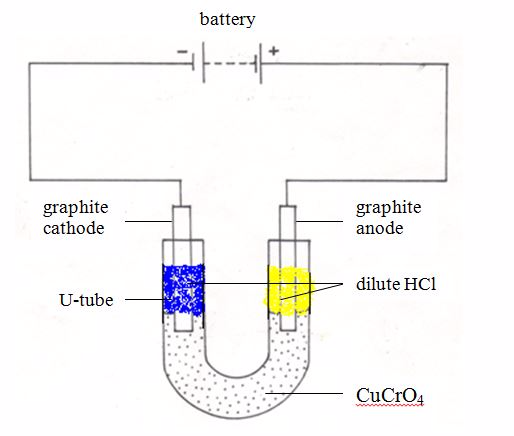Ionic Migrations During Electrolysis and the Preferential Discharge of Ions at the Electrodes
Explain ionic migrations during electrolysis and the preferential discharge of ions at the electrodes

When a salt such as sodium chloride is dissolved in water, its ions are set free to move. So the solution can be electrolysed. Since the salts, alkalis and acids are dissolved in water, most of the solutions are aqueous. There is then a complication in electrolysis of such substances in aqueous form. This is because the water used to dissolve them also do ionize partially (it is a weak electrolyte)

Then at each electrode, we get more than one ion for discharge, but only one is supposed to be discharged. Take an example of electrolysis of copper (II) sulphate solution using platinum electrodes. By ionic theory, the solution ionizes thus:CuSO4 (aq) → Cu2+ (aq) + SO42-(aq) (strong electrolyte)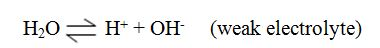During electrolysis, Cu2+ and Hions move to the cathode while SO42-and OH ions move to the anode.
 Cathode Anode Cu2+ SO42- H+ OH–

In situations like this, the order of discharge of the ions at the electrode will depend on:
1. the position of the metal ion or radical in the electrochemical series;
2. the concentration or nature of the ions (or electrolyte) to be discharged; and
3. the nature of the electrodes used.

The position of ion or radical in the electrochemical series
 Cations Anions K+ ease of dischargeincreases SO42- ease of discharge increases Ca2+ NO3– Na+ Cl– Mg2+ Br– Al3+ I– Zn2+ OH– Fe2+ Pb2+ H+ Cu2+ Ag+
The arrangement of ions above is the same as that of the electrochemical series. If all other factors are constant, any ion will be discharged from solution in preference to those above it.
 Cathode Anode Cu2+ SO42- H+ OH– Cu2+ + 2e– → Cu(s)copper is discharged (loses its charge) 4OH–→2H2O(l)+O2(g)+4e– hydroxyl ion is discharged

In other words, we can say that the reaction that occurs at the cathode is reduction (electron gain) and that which occurs at the anode is oxidation (electron loss).

Result
• At cathode, copper is deposited
• At anode, oxygen is given out (liberated)
• The solution at the end of electrolysis is colourless and acidic because in the electrolyte there are left H+ and SO42- ions, which remain moving freely in the solution as ionic sulphuric acid.

The concentration or nature of electrolyte
Take an example of electrolysis of sodium chloride using platinum electrodes when in:
1. aqueous solution;
2. concentrated aqueous solution; or
3. fused or molten state.

aqueous solution
NaCl → Na+ Cl

H2O⇔H+ + OH

During electrolysis:
 Cathode Anode Na+and H+ Cl–and OH– H+ions are discharged in preference to Na+ions: 2H+ + 2e– → H2(g) OH–ions are discharged in preference to Cl–ions:4OH–→2H2O(l) +O2(g)+4e–

Result
• At cathode, hydrogen is given out
• At anode, oxygen is out
• The concentration of the solution remains constant since all Na+ and Cl ions remain undisturbed in the solution. This means that the Na+ and Cl ions that are left in the solution are equivalent to sodium chloride solution.

Concentrated aqueous solution
NaCl → Na+ Cl

H2O⇔H+ + OH
 Cathode Anode Na+and H+H+ ions are discharged in preference to Na+ ions since Na+ and H+ are very far from each other in e. c.s2H+ + 2e– → H2(g) Cl–and OH–Cl–ions are discharged in preference to OH– ions since Cl– and OH– ions are very close to each other in the e. c. s (and because there are more Cl– ions in the solution) 2Cl–→ Cl2(g) + 2e–

Results
• At cathode H2(g) is given off
• At anode Cl2(g) is given off
• The solution becomes progressively more alkaline as the electrolysis goes on because Na+and OHions remain in solution as caustic soda (sodium hydroxide) solution. This is the main method used in the manufacture of sodium hydroxide in industry. Na+(aq) + OH(aq) → NaOH(aq)

Fused or molten sodium chloride
NaCl → Na+ Cl

During electrolysis:
 Cathode Anode Na+ + e– → Na(l) 2Cl– → Cl2(g) + 2e–

Result
• At cathode sodium is liberated (deposited)
• At anode chlorine is given off

The nature of electrodes (inert vs active electrodes)
If dilute sulphuric acid is electrolysed using platinum electrodes:

H2SO4 → 2H+ + SO42-

H2O⇔H+ + OH
 Cathode Anode 2H+ + 2e– → H2(g) SO42- and OH– 4OH–→2H2O(l)+O2(g)+4e–

Result
• At cathode H2(g) is given out
• At anode O2(g) is given out
• The solution becomes acidic at the end of electrolysis because of the acidic ions (SO42-) left in the finala solution.

Charge flow during electrolysis
The coulomb is the electrolytic unit of charge. A current of one ampere is the rate of flow of charge equal to one coulomb per second.The charge is calculated from the knowledge of the number of seconds for which a steady current is passed.
 Current in circuit Time taken Total charge 1 ampere 1 second 1 coulomb 1 ampere 10 seconds 10 coulombs 20 amperes 10 seconds 200 coulombs A amperes t seconds At coulombs

Therefore charge = quantity of electricity (Q) = I×t

Flow of charge required to liberate 1 mole of element during electrolysis
Electrolysis always produces chemical reactions. Consider a reaction (at cathode) in which one mole of silver Ag+ ions is discharged and deposited.Ag+ + e– → Ag(s)

In this case, 1 mole of electrons (e) is required to discharge 1 mole of Ag+ ions to produce 1 mole of silver atoms (Ag(s)). 1 mole of electrons is a large charge and experiments show that it is equal to 96500 coulombs. Therefore 1 mole of electrons = 96500 coulombs. This is called the faraday.

The number of faradays (moles of electrons) required to liberate 1 mole of an element during electrolysis is deduced from the equation for the electrode reaction.

Example 1

 Element Electrode reaction Faradays Sodium Na+ + e– → Na(s) 1 Copper Cu2+ + 2e– → Cu(s) 2 Aluminium Al3+ + 3e– → Al(s) 3 Chlorine 2Cl– → Cl2(g) + 2e– 2

That is, 1 faraday is needed to deposit 1 mole of sodium atoms (23g), 3 faradays to deposit 1 mole of aluminium atoms (27g) and 2 faradays to liberate 1 mole of chlorine gas (71g).
• The mass of an element liberated by 1 coulomb of electricity during electrolysis is called electrochemical equivalent of that element.
• The mass of an element deposited or liberated by 1 faraday during electrolysis is called chemicale quivalent of that element.

Experiments to Relate Masses Liberated and Quantity of Electricity Passed
Carry out experiments to relate masses liberated and quantity of electricity passed
The laws expressing the quantitative results of electrolysis were first stated by a British chemist called Michael Faraday. The laws assert that the amount (expressed in moles) of an element liberated during electrolysis depends on:
1. the time of passing the steady current;
2. the magnitude of the steady current passed; and
3. the charge on the ion of the element.

An Experiment to Verify Faraday’s First Law of Electrolysis
Carry out an experiment to verify Faraday’s First Law of Electrolysis
The fact that the amount of a substance liberated during electrolysis depends upon these factors can be proved by conducting experiments. The product of time (measured in seconds) and the current passed (measured in amperes) gives a measure of electricity known as the quantity of electricity.

Quantity of electricity (Q) [coulombs]= current (I) [amperes]×time (t) [seconds]

Q = I × t

Because of this relationship, factors (1) and (2) may be included in the same experiment. The experiment to determine the effect of time on the amount of element deposited or liberated is carried out by passing a steady current through a solution of the compound of that element for different lengths of time.

The following table summarises the specimen results obtained by passing a steady current (0.21 amps) through a solution of copper (II) sulphate for 15, 30, 45 and 60 minutes. The last column shows the mass of copper deposited.
Specimen results for electrolysis of copper (II) sulphate
 Current (amps) Time(s) Quantity of electricity (coulombs) Mass of copper deposited (grams) 0.21 15 × 60=900 900 ×0.21=189 0.063 0.21 30 × 60=1800 1800 ×0.21=378 0.129 0.21 45 × 60=2700 2700 ×0.21=576 0.187 0.21 60 × 60=3600 3600 × 0.21=756 0.250

The relationship between the amount of copper deposited and the quantity of electricity passed can be assessed by considering the values in the last two columns in the table. This data may be represented in a graph illustrated in figure 6.4. The shape of the graph shows a straight line passing through the origin. From this fact, it is clear that the mass of copper deposited is directly proportional to the quantity of electricity passed. This is in accordance to what Faraday formulated in his First Law.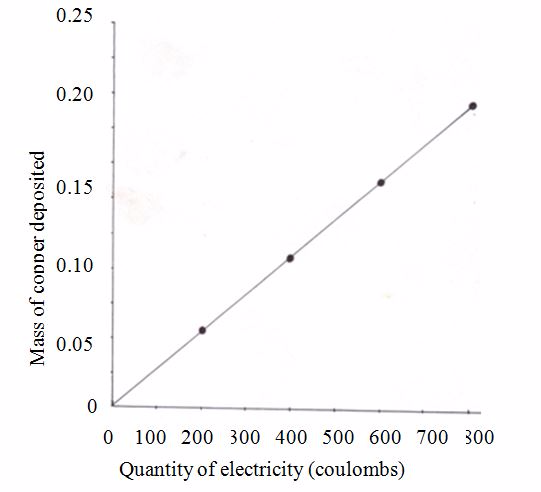Graph of mass deposited versus amount of electricity passed

Faraday’s First Law of Electrolysis states that the mass of a substance liberated at (or dissolved from) an electrode during electrolysis is directly proportional to the quantity of electricity passing through the electrolyte.

The quantity of electricity is measured in coulombs where a coulomb is the passage of an electric current of one ampere for one second. Let
• m be the mass of the substance liberated;
• be the current passed in amperes; and
• t be the time in seconds.

We can therefore represent the first law mathematically as:m α I × t or m = Z × I × t where Z is the proportionality constant referred to as electrochemical equivalent of the substance liberated. Electrochemical equivalent is the mass of a substance (element) liberated by 1 coulomb of electricity during electrolysis.

An Experiment to Verify Faraday’s Second Law of Electrolysis
Carry out an experiment to verify Faraday’s Second Law of Electrolysis
The third (3) factor mentioned previously as affecting the amount of substance liberated during electrolysis may also be investigated experimentally. Because our interest is the effect of the charge on the ions present in solution, we need to keep the quantity of electricity fixed whilst varying the types of the ions in solution. This may be achieved by passing the same quantity of electricity through two cells, with ions of different charges in each cell.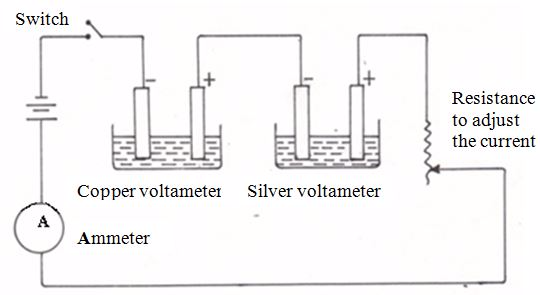Apparatus for confirmation of Faraday’s Second Law

The experiment is conducted using two voltameters. The two voltameters are connected in series as shown. The first one is a copper voltameter and the second is a silver voltameter.The copper voltameter has copper electrodes in a solution of copper (II) sulphate. Hence, in this voltameter, copper ions are discharged and deposited at the cathode.

The silver voltammeter has silver electrodes in a solution of silver nitrate. The discharged silver ions are deposited at the cathode.

Before the experiment is started, each cathode electrode in the voltameters is cleaned, dried and weighed. The electrodes are then connected to the circuit, after which a suitable current is passed for a measured period of time.

After this, the cathode are removed from the voltameters, cleaned, dried and reweighed. The increase in mass of the two cathode electrodes represents the respective amounts of copper and silver deposited at the cathodes. The quantity of electricity required to deposit one mole of each element is calculated using Faraday’s Second Law of Electrolysis.

Specimen results
 Current flowing = 0.45A Duration of current flow = 25 minutes Mass of copper deposited = 0.221g Mass of silver deposited = 0.755g

The results show that the masses of silver and copper deposited are different. A comparison of the amounts of each of the elements deposited can be made simple by calculating the number of moles of atoms of each of the element deposited.

Thus:

Amount of copper deposited = 0.221/63.5mole = 0.0035 mole

Amount of silver deposited = 0.755/107.8mole = 0.0070 mole

It is seen that twice as many atoms of silver are deposited as atoms of copper. The difference in amount of each element deposited arises from the difference in charges on ions of the element concerned.

The change on the copper ion is twice that on the silver ionand therefore twice the quantity of electricity will be required to liberate one mole of copper as for the liberation of one mole of silver.

This relationship is in accordance to Faraday’s Second Law of Electrolysis, which describes the relationship between the amount of element deposited and the charge on the ions of that element. Faraday’s Second Law of Electrolysis states that when the same quantity of electricity is passed through solutions of different electrolytes the relative numbers of moles of the elements deposited are inversely proportional to the charges on the ions of each of the elements respectively.

In order to discharge one mole of monovalent ions such as hydrogen ion, Sodium ion, Silver ion and Chlorine ion,96500 C of electricity are required. This quantity of electricity has been experimentally determined and is known as the Faraday constant.

It represents one mole of electrons, which is the same as the quantity of electrons required to discharge one mole of Siliverions to give one mole of silver atom. The validity of Faraday’s Second Law of Electrolysis is evident from the following observations:
1. One faraday (IF) discharges one mole of H+, Na+, Ag+, Cl and OH– ions.
2. Two Faradays (2F) discharges one mole of Cu2+, Pb2+, Mg2+, Ca2+, Fe2+, etc ions.
3. Three Faradays (3F) discharge one mole of Al3+, Fe3+, etc. ions.

Relationship between the Chemical Equivalents of Elements and Quantity of Electricity Passed
Relate the chemical equivalents of elements and quantity of electricity passed

A steady current of 4 amperes is passed through aqueous copper (II) sulphate solution for 1800 seconds using platinum electrodes. Calculate:
1. mass of copper deposited
2. mass of oxygen liberated

Given:
• Atomic weight of copper = 63.5
• Atomic weight of oxygen = 16
• 1 Faraday = 96500 C

Solution

Cathode reaction: Cu2+ + 2e → Cu(s)

In this case, 2 Faradays of electricity are required to deposit one mole of copper atom 63.5g.

This means 2 × 96500 C liberates 63.5g of copper.

Quantity of electricity passed = I×t = 4×1800C. So, if 2×96500 C liberates 63.5g, thenI×t = 4×1800Cwill liberate2.4g of copper.

Therefore, mass of copper deposited = 2.4g

Anode reaction: 4OH→2H2O(l) + O2(g) + 4e

The reaction shows that 4 moles of electrons (4 Faradays) are lost during the reaction process.

Therefore, 4 × 96500 C are needed to liberate one mole (32g) of oxygen.

Quantity of electricity flowing = 4 × 1800 C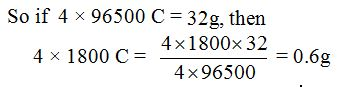Therefore, mass of oxygen liberated = 0.6g

Electrolysis has several uses in industry. Its main application has been in the fields of manufacture of chemicals and in the purification of metals for which other purification methods prove either too difficult or highly expensive to apply. Some applications of electrolysis are as discussed below:

The Industrial Purification of Copper by Electrolysis
Outline the industrial purification of copper by electrolysis

Some metals can be purified by means of electrolysis. This process is used in industry to purify copper, which must be very pure 99.9% for electrical wiring. Copper made by roasting the sulphide ore is about 99.5% pure (so it has an impurity level of 0.5%). This level of impurity cuts down electrical conductivity significantly.

This is how the electrolytic purification (refining) process is carried out:The anode is made of a large block of impure copper. The cathode is a thin sheet of pure copper. The electrolyte is copper (II) sulphate solution.During the refining process, the copper atoms of the impure block become ions (the anode dissolves).Cu → Cu2+ + 2e

The ions from the solution become atoms.

Cu2+ + 2e → Cu(s)

They stick onto the cathode. A layer of pure copper builds up on the cathode. As electrolysis takes place, the cathode gains mass as copper is deposited on it. As a result, the cathode gets smaller while the cathode gets bigger as electrolysis proceeds. Eventually the whole cathode dissolves.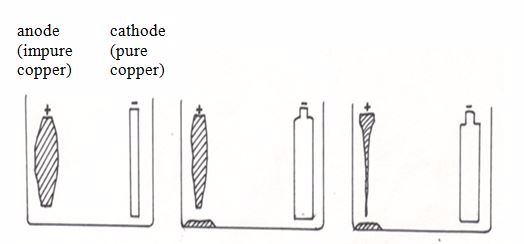Purification of copper by electrolysis

Only pure copper sticks to the cathode. Most impurities fall to the bottom of the electrolytic cell. They form a solid material (anode sludge or slime) which contains small quantities of precious metals such as silver, gold and platinum. The precious metals recovered from the slime are purified and sold.

An Experiment on Electroplating of Metallic Materials
Carry out an experiment on electroplating of metallic materials
Electroplating is the coating of a metal with a layer of another metal by means of electrolysis. Electrolysis can be used to coat a thin layer of a less reactive metal onto a more reactive metal. The thin layer of less reactive metal will provide protection from corrosion for the more reactive metal underneath. It may also make the product more attractive.

The object to be coated should be made the cathode and the coating material should be the electrolyte. The most commonly used metals for electroplating are copper, chromium, silver and tin.

Steel can be electroplated with chromium or tin. This prevents the steel from rusting and gives it a shiny, silver finish. This is also the idea behind chromium-plating articles such as car bumpers, kettles, bath taps, etc. Chromium does not corrode, it is a hard metal that resists scratching and wear, and can also be polished to give an attractive finish.

Nickel can be electroplated with silver. This will make nickel more attractive.The diagram below shows how a steel jug is electroplated with silver. The jug becomes the cathode of an electrolytic cell. The anode is made of silver. The electrolyte is a solution of a silver compound, for example silver nitrate.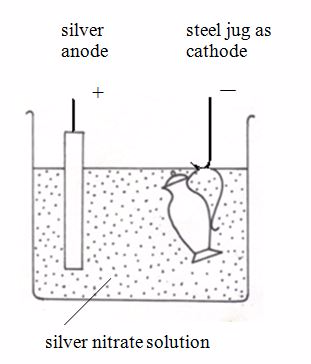Silverplating a steel jug

At the anode: The silver dissolves, forming ions in solution:Ag → Ag+ + e

At the cathode: The silver ions receive electrons, forming a coat of silver on the jug: Ag+ + e→Ag (s)

When the layer of silver is thick enough, the jug is removed.In general, to electroplate any object with metal M, the set up is:
• Cathode – object to be electroplated
• Anode – metal M
• Electrolyte – solution of a soluble compound of M

Select the fields to be shown. Others will be hidden. Drag and drop to rearrange the order.
• Image
• SKU
• Rating
• Price
• Stock
• Availability